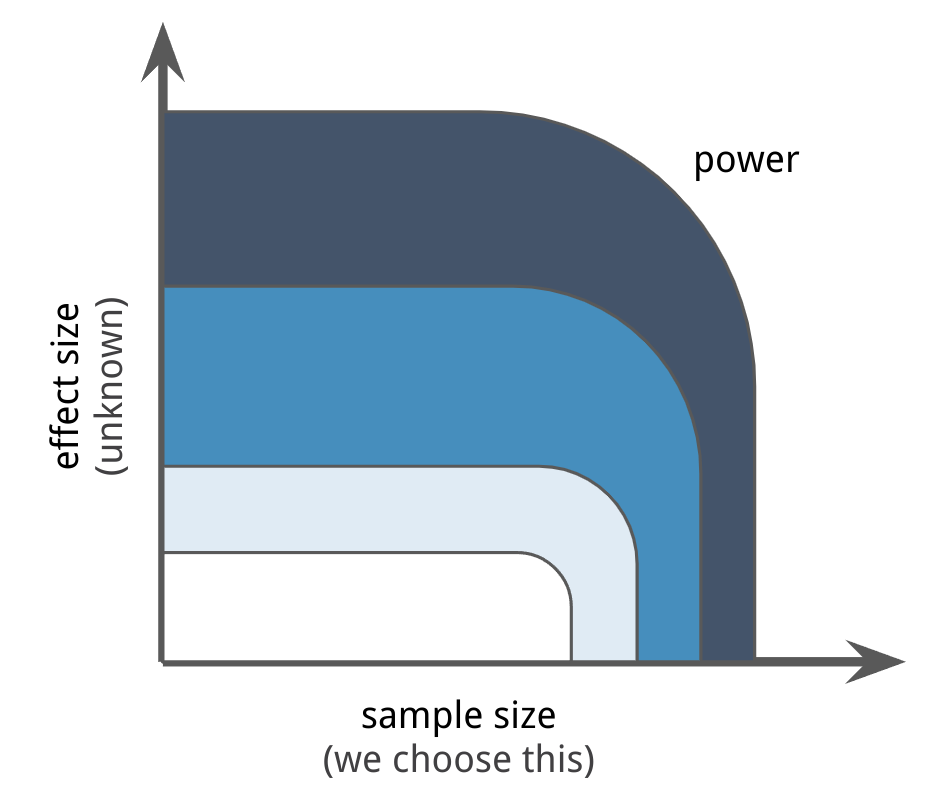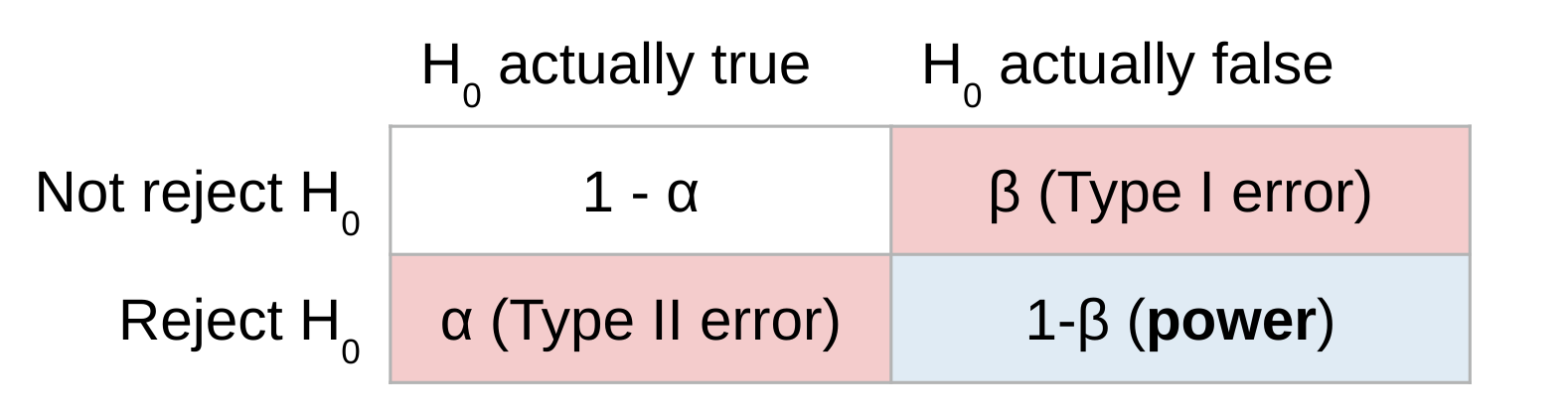### Bayesian equivalent of power analysis

This question has come up a fair amount recently, and I've found myself trying to dig up a comment I wrote on an old, since-deleted Reddit post. Copying it here so it's easier to find in the future.

Just like we use simulation to see if our models can effectively recover the parameters of simulated data, we can use simulations to see if our models would have effectively led to the right answer given certain values of sample size (often under your control, though potentially expensive) and effect size (unknown).

As in frequentist analysis, one being large will often make up for the other being small, but if you want to know what frontier will lead you to draw correct conclusions, you'd want to test that for realistic combinations of sample size and effect size.You're going to want to look up the terms "region of practical equivalence" (ROPE) and Kruschke's writing about power analysis.1 2 Also excellent is Gelman, Hill, and Vehtari's book Regression and Other Stories which covers this in chapter 16 ("Design and sample size decisions").3

Another nice thing about the Bayesian approach here is that if you can encode your decision policy downstream of this inference or quantify the practical consequence of whatever "false positive" or "false negative" means in your case, you can actually just layer that on to your simulated scenarios and quantify what would have happened.

For example: "if the sample size is $N$ and the true parameters we're trying to uncover are actually $y$, we would end up inferring that the parameters are $\hat y$ which would result in outcome X" and also "our prior belief about the true parameters is $\pi$ meaning that, over all possible outcomes for $\hat y$, we would expect outcome Z"...1 Kruschke, J. (2013). Bayesian estimation supersedes the t test. Journal of Experimental Psychology: General, 142(2), 573–603. doi:10.1037/a0029146

2 Kruschke, J., & Liddell, T. M. (2017). The Bayesian New Statistics: Hypothesis testing, estimation, meta-analysis, and power analysis from a Bayesian perspective. Psychonomic Bulletin & Review. doi:10.3758/s13423-016-1221-4

3 Gelman, A., Hill, J., & Vehtari, A. (2020). Regression and Other Stories (Analytical Methods for Social Research). Cambridge: Cambridge University Press. doi:10.1017/9781139161879

Any comments or suggestions? Let me know.